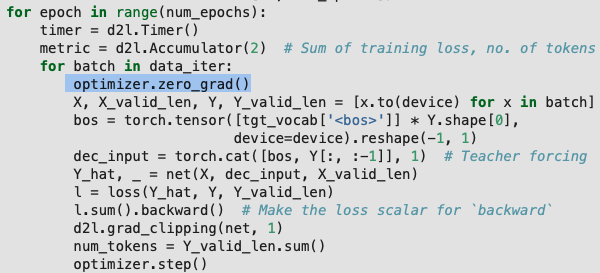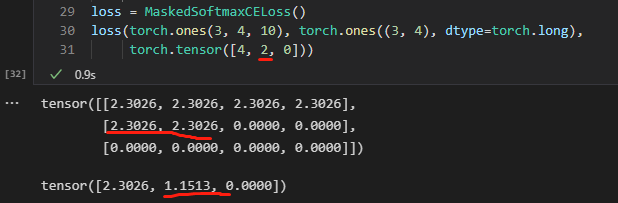# 序列到序列学习（seq2seq）

9.7.5 的预测代码中

5 Likes

train_seq2seq 函数的训练 step 中是不是需要 optimizer.zero_grad() ?Moon 指的是 context 在变化，这是个问题。

2 Likes

``````def bleu(pred_seq, label_seq, k):  #@save
"""计算 BLEU"""
pred_tokens, label_tokens = pred_seq.split(' '), label_seq.split(' ')
len_pred, len_label = len(pred_tokens), len(label_tokens)
score = math.exp(min(0, 1 - len_label / len_pred))
for n in range(1, k + 1):
num_matches, label_subs = 0, collections.defaultdict(int)
for i in range(len_label - n + 1):
label_subs[''.join(label_tokens[i: i + n])] += 1
for i in range(len_pred - n + 1):
if label_subs[''.join(pred_tokens[i: i + n])] > 0:
num_matches += 1
label_subs[''.join(pred_tokens[i: i + n])] -= 1
score *= math.pow(num_matches / (len_pred - n + 1), math.pow(0.5, n))
return score
``````

I think the code above should be corrected to:

``````def bleu(pred_seq, label_seq, k):  #@save
"""计算 BLEU"""
pred_tokens, label_tokens = pred_seq.split(' '), label_seq.split(' ')
len_pred, len_label = len(pred_tokens), len(label_tokens)
score = math.exp(min(0, 1 - len_label / len_pred))
for n in range(1, k + 1):
num_matches, label_subs = 0, collections.defaultdict(int)
for i in range(len_label - n + 1):
label_subs[' '.join(label_tokens[i: i + n])] += 1
for i in range(len_pred - n + 1):
if label_subs[' '.join(pred_tokens[i: i + n])] > 0:
num_matches += 1
label_subs[' '.join(pred_tokens[i: i + n])] -= 1
score *= math.pow(num_matches / (len_pred - n + 1), math.pow(0.5, n))
return score
``````

the space is needed here.
for example “ad og” is different from “a dog” in bigram.

2 Likes

Thanks @howardchina, nice catch. This will be fixed in master now.

1 Like

##运行结果不一样，有没有大神能给解释一下

import torch
from torch import nn
torch.manual_seed(1)

X = torch.rand(2, 3, 4)
flatten=nn.Flatten()
X = flatten(X)
Y = X
print(‘X.shap’, X.shape)
layer=nn.Linear(12,2)
x = layer(X)
print(‘x:’, x)
net1 = nn.Sequential(nn.Linear(12, 2)
)
y = net1(Y)
print(‘y:’, y)

2 Likes
2 Likes

hidden_state有指定吧， hidden_state不就是输入参数里面的state吗，这里的state应该不只是加到输入上，而且应该包含了将state作为隐变量进行向前传播。

1. 既然有效长度是2， 为什么还要求平均，不应该是求和除以2吗，第二个元素也应该是2.306